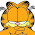## 2014/11/06

### Printing function calls in Perl

Lately my favourite way to interpolate function calls into printed strings is to use the \${\ ... } notation for embedding arbitrary expressions into strings:

`print "f(\$number) = \${\ fibonacci(\$number) }\n";`

Other techniques include separate arguments:

`print "f(\$number) = ", fibonacci(\$number), "\n";`

or a helper variable:

```my \$result = fibonacci(\$number);
print "f(\$number) = \$result\n";```

or even printf:

`printf "f(%d) = %d\n", \$number, fibonacci(\$number);`

Of all these techniques I tend to prefer either of the first two, because they lead to putting the expressions "in-line" with the rest of the text string, whereas in the latter two they sit elsewhere, making it harder to see at a glance what gets printed where. Especially with printf's positional arguments, it can be easy to be "off-by-one" with a large number of arguments, and put everything in the wrong place.

In addition, the first technique is not limited to print calls, as it works just on simple string interpolation, so it works just as well to construct strings in general.

(This post was originally written to answer a question on StackOverflow.)

#### 5 comments:

1.Personally I prefer the symmetry of the babycart operator: @{[…]}

2.I prefer the printf/sprintf approach in general as I think it is easier to read in the long term... and makes for more readable code in the long term...

3.Can you please elaborate more on the \${\ } notation?
A Perl newbie...

4.Second that. What is \${\ }?

5.Regarding \${ \.. } check "How do I expand function calls in a string?" in perlfaq4